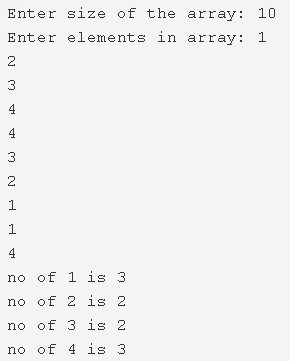# C Program To Count Frequency Of Each Element In Array | C Programs

C program to count the frequency of each element in an array – In this article, we will detail in on the several means to count the frequency of each element in an array in C programming.

Suitable examples and sample programs have also been added so that you can understand the whole thing very clearly. The compiler has also been added with which you can execute it yourself.

The means used in this piece are as follows:

• Using Standard Method
• Using FunctionFirstly, enter the size of the array that you are concerned with.

The array size, in this case, is 10.

With that, you need to enter the elements of the array as well.

The elements entered in this array are as follows:

1 2 3 4 4 3 2 1 1 4

You can see the frequency can be easily seen.

• No. of 1s: 3
• No. of 2s: 2
• No. of 3s: 2
• No. of 4s: 3

Thus, the methods used in this piece are as follows:

## Using Standard Method

1. Read the entered array size and store that value into the variable n.

2) Read the entered elements and store the elements in the array a[] as scanf(“d”,&a[i]) using for loop for(i=0;i<n;i++).

3) for loop iterates from i=0 to i<n,

a) if a[i]!=-1

a.1) Compare each element with remaining elements of the array as a[i]==a[j] using for loop from j=i+1 to j<n.

a.2) If any two element are equal to each other then increase the count value by 1.

a.3) a[j]=-1.

b) Store the count value at b[i].i.e b contains the count numbers of each element of the array.

4) Print the each element along with their count number as printf(“no of %d is %d \n”,a[i],b[i]) using for loop from i=0 to i<n.Here Print Method

Output:

### Using Function

1. The main() function calls the count() by passing array a, empty array b, the size of the array n are as arguments to the function count().

2) In Count function

for loop iterates through i=0 to i<n

if a[i]!=-1

a) Compare present element with next elements of the array using for loop from j=i+1 to j<n.

If any element is equal to the present element then increase the count by 1. Repeats until all iterations of j.

b) Store the count value into b[i]. Repeat this step until all iterations of i.

3) The main() calls the print() function by passing array a, the count array b, size of the array is as arguments. The print function prints the array elements along with their count value.

Output:
x

## C Program To Print Number Of Days In A Month | Java Tutoring

C program to input the month number and print the number of days in that ...